# Bra-ket notation

Bra-ket or bracket (or even bra-c-ket) notation was formulated by Dirac to provide a concise method for performing and describing the linear algebra used throughout the matrix mechanics formulation of quantum mechanics. The notation is in wide use in the field today, and although developed with quantum mechanics in mind it can be employed more generally when working with any vector space. In this notation vectors are represented by kets, such as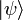$|\psi\rangle$, while their corresponding dual vectors are given by bras,$\langle\psi|$. In the context of quantum mechanics the state of a system corresponds to a vector in a Hilbert space, so the state$|\psi\rangle$ is analogous to the wave function ψ(x).

### Mathematical description

Let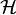$\mathcal{H}$ be a Hilbert space and$\mathcal{H}^*$ its dual space (which is isomorphic to$\mathcal{H}$ if the space is finite-dimensional). Elements of$\mathcal{H}$ are then labelled by kets and elements of$\mathcal{H}^*$ are labelled by bras. Together a bra and a ket can form a Dirac bracket,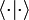$\langle\cdot|\cdot\rangle$, which is equal to the inner product between them. The bracket then is a map from$\mathcal{H}^*\times\mathcal{H}$ to a field F (in quantum mechanics the field is the complex numbers,$\mathbb{C}$).

When the order of the bra and ket is reversed the resulting object is an operator, sometimes called a ket-bra,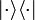$|\cdot\rangle\langle\cdot|$. This operator is given by the outer product of the ket with the bra, and is a map from$\mathcal{H}$ onto itself since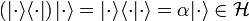$\left(|\cdot\rangle\langle\cdot|\right)|\cdot\rangle =|\cdot\rangle\langle\cdot|\cdot\rangle=\alpha|\cdot\rangle \in\mathcal{H}$, where$\alpha=\langle\cdot|\cdot\rangle\in F$ is a scalar. By convention, duplicated vertical bars in an expression are dropped as we have done here (i.e. writing$\langle\cdot|\cdot\rangle$ instead of$\langle\cdot||\cdot\rangle$).

## Uses in quantum mechanics

Suppose that$\mathcal{H}$ corresponds to the state space for a quantum system. For example, if the system was a particle in a box then$\mathcal{H}$ would contain every possible state that the particle could occupy. Now let the state of the system be$|\psi\rangle\in\mathcal{H}$, with$|\psi\rangle$ normalized (that is,$\langle\psi|\psi\rangle=1$) and let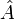$\hat{A}$ be an operator corresponding to the observable A.

Expectation value

The expected result of a measurement of A is given by$\langle\hat{A}\rangle=\langle\psi|\hat{A}|\psi\rangle$.

Overlap and probability

The overlap between the state of the system and another state$|\phi\rangle\in\mathcal{H}$ is$\langle\phi|\psi\rangle$, which means that the probability of finding the system in state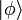$|\phi\rangle$ is given by$|\langle\phi|\psi\rangle|^2$. This can also be seen as the expectation value of the projection operator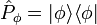$\hat{P}_\phi=|\phi\rangle\langle\phi|$, since this yields$\langle\hat{P}_\phi\rangle =\langle\psi|\phi\rangle\langle\phi|\psi\rangle =\langle\psi|\phi\rangle\langle\psi|\phi\rangle^* =|\langle\phi|\psi\rangle|^2$

Resolution of the identity

If the states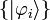$\{|\varphi_i\rangle\}$ are the (normalized) eigenstates of$\hat{A}$ then the identity operator can be expressed as$I=\sum_i|\varphi_i\rangle\!\langle\varphi_i|$.

This result holds if the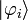$|\varphi_i\rangle$ are any complete set of orthonormal vectors, which is guaranteed to be the case for the eigenvectors of a Hermitean matrix.